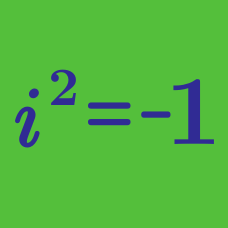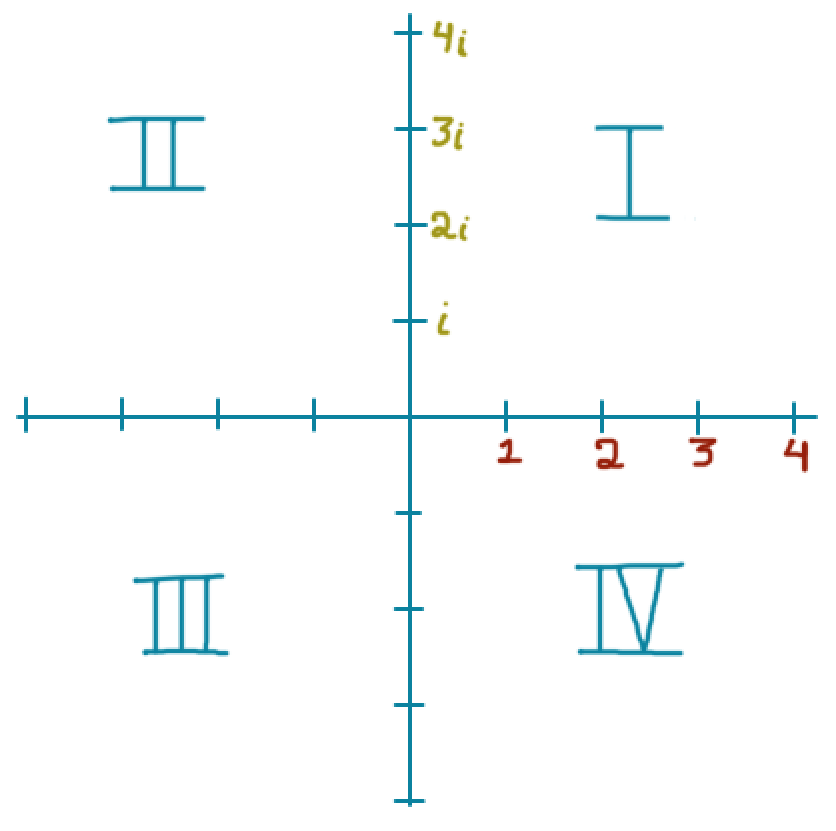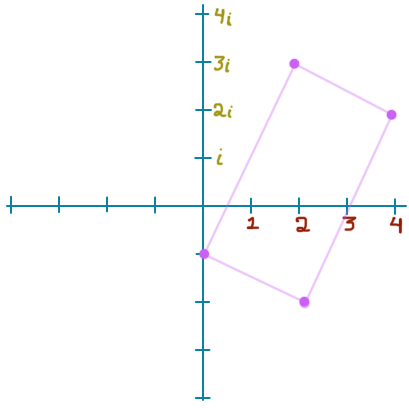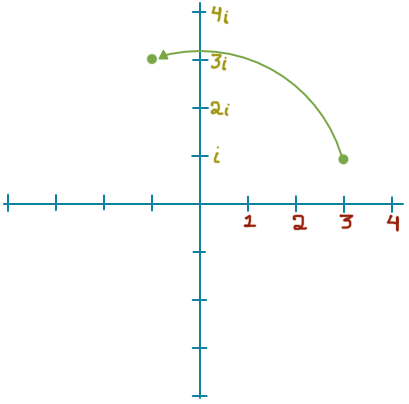Algebra

# Complex Numbers - Argand PlaneSuppose that we have two complex numbers $z_1 = 3 - 2i$ and $z_2 = 4 - 3i$.

In what quadrant is this complex number $z_1z_2$ located?

What is the shape of graph of the equation $\left|z\right| = 4$ in the complex plane?In $\text{units}^2$, what is the area of the rectangle formed by the vertices $2 + 3i, 4 + 2i, -i$, and $2 - 2i$?Suppose the function $f(z)$ takes the complex number $z$ and rotates it 90 degrees counterclockwise around the origin.

Which function represents this transformation?

For a complex number $z$, on which part of the Argand plane does $\text{Re}(z) + \text{Im}(iz)$ sit?

×